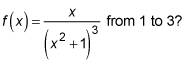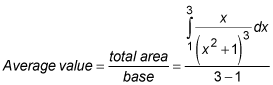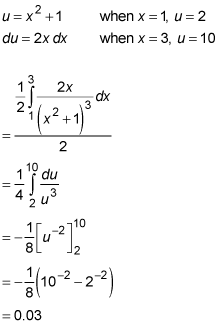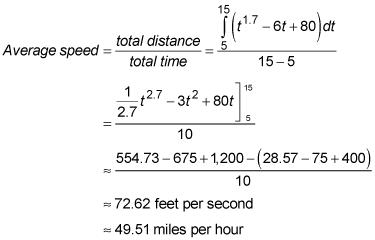##### Differential Equations For DummiesAlthough differentiation helps you figure out a lot of things about a function, there's one thing that differentiation cannot answer: What's the function's average height? To answer that, you need integration.

The following practice questions ask you to find average values using integration.

## Practice questions

1. What's the average value of2. A car's speed in feet per second is given by f(t) = t1.7 – 6t + 80. What's its average speed from t = 5 seconds to t = 15 seconds? What's that in miles per hour?

## Answers and explanations

1. The average value is 0.03.Do this with a u-substitution.2. The car's average speed is about 72.62 feet per second, or 49.51 miles per hour.